Updated on 26 Jul 2021

# Working With Loops in PythonMartin Ganchev
Published on 24 Jul 2018 13 min read

The main reason we need loops in Python is that it is way easier to let the program go through a certain block of code a few times rather than writing it repeatedly ourselves. This is one of the great advantages programming offers.

In the example we will be giving, we will be using a list, so if you are not sure what it is, please get acquainted with them with this tutorial.

Iteration is a fundamental building block of all programs. It is the ability to execute a certain code repeatedly. In this tutorial, we will focus on a few examples of iteration processes in Python.

## Python For Loop: Explanation and Examples

To begin, we have prepared a list, called even. It contains all the even numbers from 0 to 20.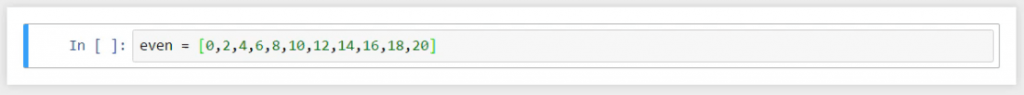Imagine we want these numbers printed out. So, we write the following

for n in even:
print(n)

which would mean for every element n in the list even, print n. In this case, n is called the loop variable. We are not required to call it n; any other name would work fine.

### The Body of For Loops in Python

The phrase print n acts as the body of our loop. Don’t forget, to run the loop properly in Python it should be indented.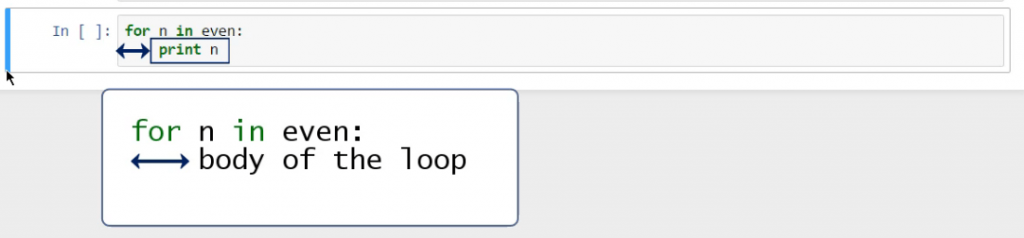The command in the loop body is performed once for each element in the even list. Now, let’s go over the steps implied by this piece of code.

### What's the Process Behind For Loops?

The loop starts by taking an element n from our list. Then, the computer executes the body of the loop. In our case, it will simply print that variable.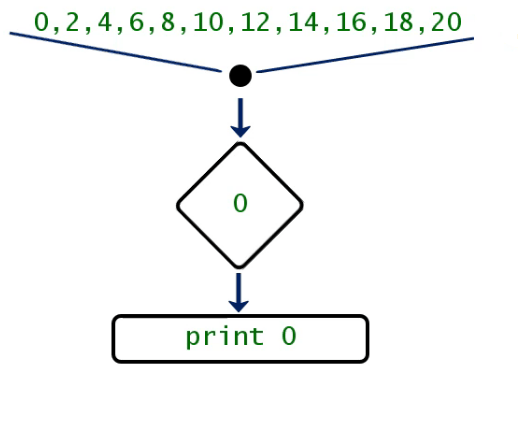When the computer is done with this operation, called iteration (or pass) of the loop, Python will go back to the for statement and pick the next element n that is in the even list.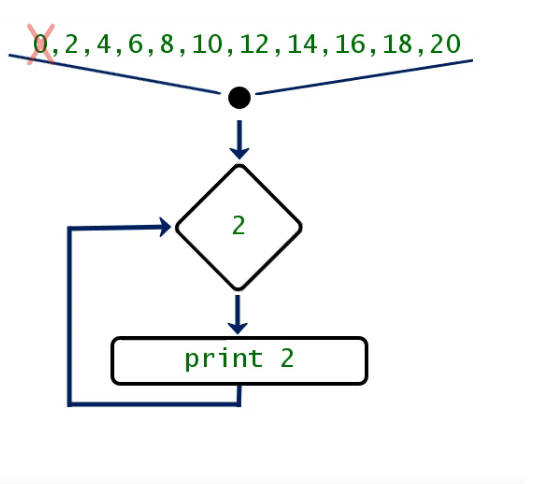It will then print it out and so on and so forth until the loop body has been executed for all available elements in the list.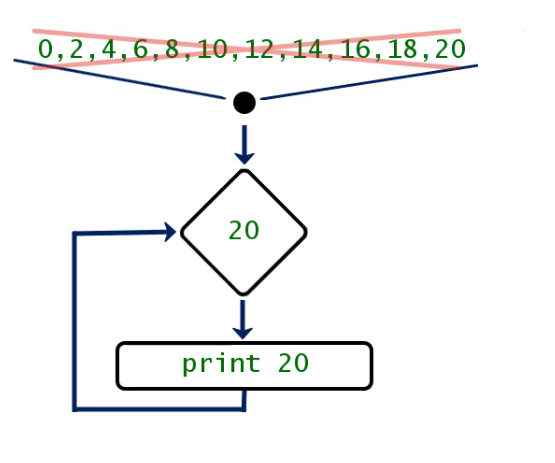So, let’s see what the output of this code is: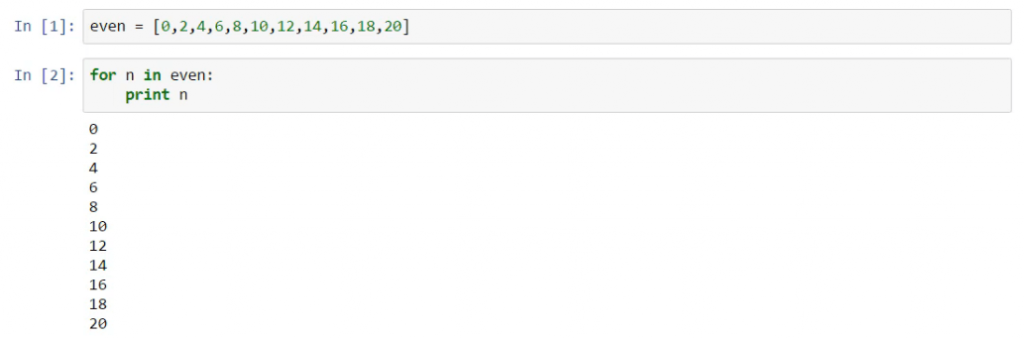Exactly as expected, all numbers from the list are printed out in a column.

### How to Print Elements on a Single Row?

What if we wanted to see them ordered in a single line? A comma after the element n in the command “print n” will achieve that. The comma will indicate every next element from the loop should be placed on the same row.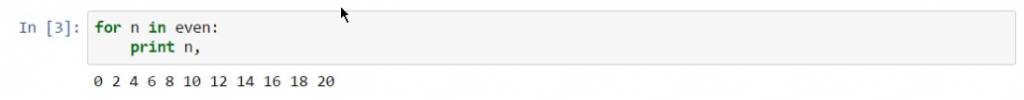### How to Create Loops with range()?

Another example of the brilliance of the loops in Python is how easily we can print all powers of 2. To print all the values from 20 to 29, we can use the following code:

for n in range(10):
print (2 ** n)

I guess you must agree that it was not necessary to specify the name of a list that exists in our code – using a list created through the range() function is going to work too!

Note: Range (x) creates a list of all integers from 0 to X-1. For instance, range(10) as in the example would create the list [0,1,2,3,4,5,6,7,8,9]. The range function is mainly used for iterating over loops.

## Python While Loop: Explanation and Example

The same output we obtained earlier could be achieved by using a while loop, instead of a for loop.

However, the structure is slightly different.

Initially, we will set a variable x = 0. And we’ll say: while this value is smaller than or equal to 20, print x.

x = 0
while x<=20:
print(x)

Important: If you leave the code like this, you will run into an infinite loop, and your computer will crash!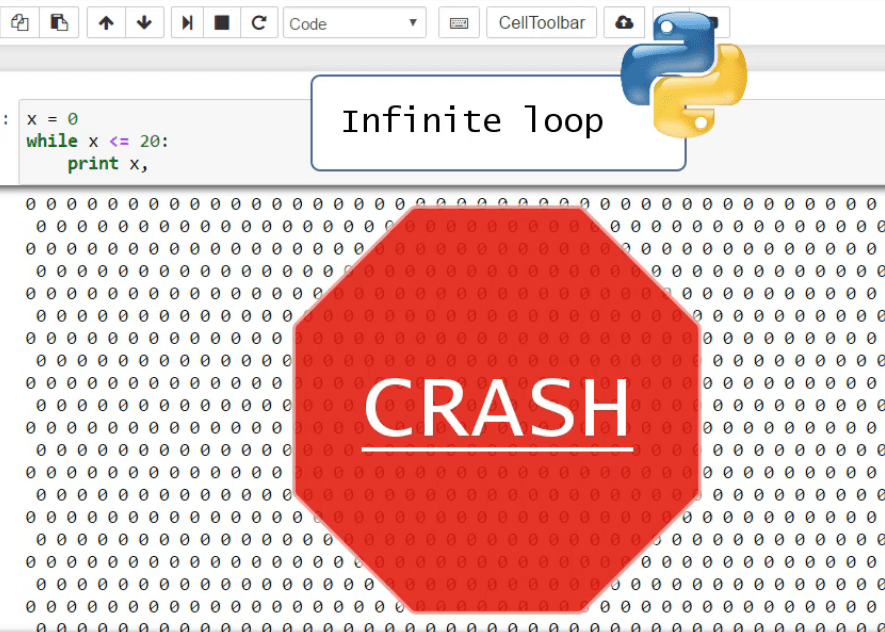So be very careful; since x will always be smaller than 20, your loop will be infinite. It will iterate the same variable repeatedly. This is what we did here with x = 0.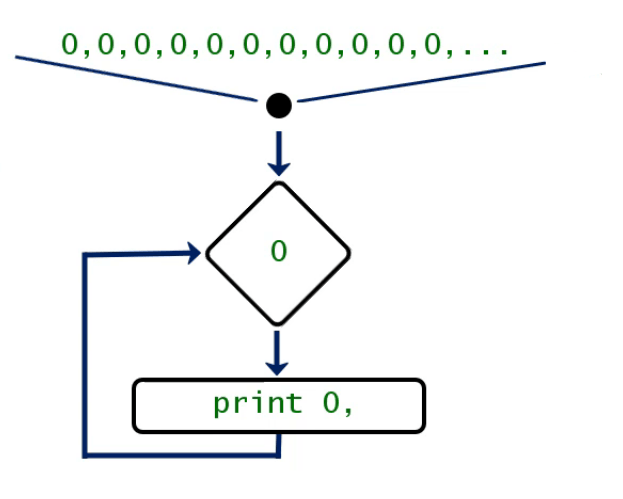### The Body of While Loops

And “always” is not what we want in an iteration. We want the loop to end. The way we can achieve that is by adding a line of code that specifies a change in x or what must happen to x after it is printed. In our case, we will tell the computer to bind x to a value equal to x + 2.

### What is Incrementing?

Actually, there is a term for what we just did. In programming terms, adding the same number on top of an existing variable during a loop is called incrementing.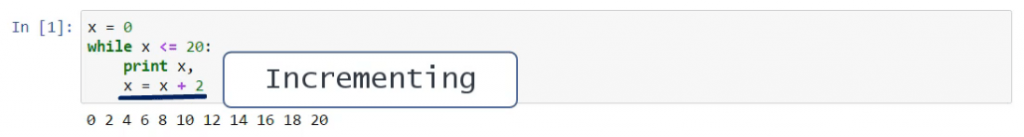The amount being progressively added is called an increment. In our case, we have an increment of 2.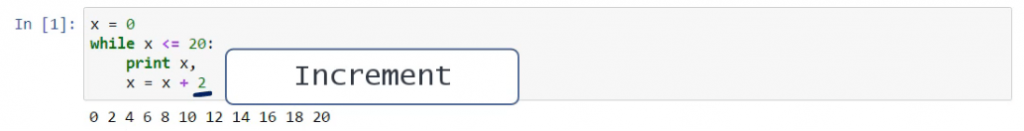### Another Way to Increment

Furthermore, the Pythonic syntax (similar to other programming languages) offers a special way to indicate incrementing. “x += 2” shows we are incrementing the value of 2 on top of the base x, just as if we had typed “x = x + 2”. As you can see in the picture below, the two outcomes are the same.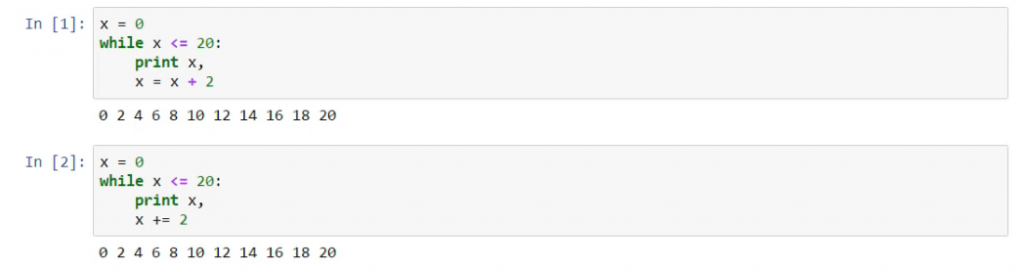## Python Conditional Statements and Loops

Now, let’s be brave and create an iteration that includes a conditional in the loop body. We can tell the computer to print all the even values between 0 and 19 and state “Odd” in the places where we have odd numbers.

for x in range(20):
if x % 2 == 0:
print(x)
else:
print('Odd')

Let’s see how it actually works.

1 - If x leaves a remainder of 0 when divided by 2, which is the same as to say “if x is even”, print x on the same line.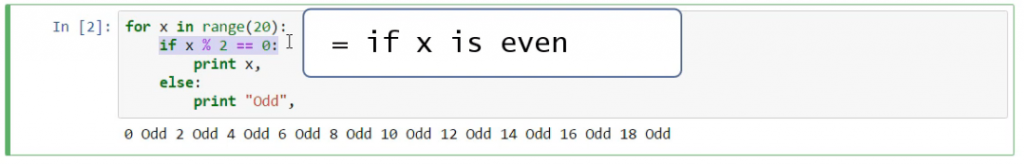2 - “Else”, which means unless x is even, or if x is odd, print “Odd”.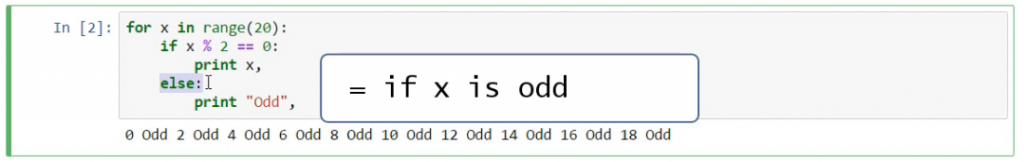So now you know that there is nothing scary about using conditionals in the body of a loop. Well, except the double indentation.

## Another Way to Program Loops in Python

There are two main ways to program a loop, and we only looked at the second one once. Let’s go over it one more time.

We have a list x that contains the numbers 0, 1, and 2. [

x = [0, 1, 2]

We saw we can print out each of its elements by typing

for item in x:
print(item)

The second way finds its practical application in more sophisticated codes. Its structure takes advantage of the range() and len() functions in the following way:

for item in range(len(x)):
print(x[item])

If we do this, the variable item will loop through a new list created by range(), and that has as many elements as the x list itself.

len() is a function which returns the length of a given object. X is a list with 3 elements, thus, len(x) = 3.

Range() is a function with three arguments: range ([start], stop, [step]), where only the end point argument is mandatory. From above, we know that:

Range(3) = [0,1,2]

Range(10) = [0,1,2,3,4,5,6,7,8,9]

If we want to include starting point, we can do so.

Range(5,10) = [5,6,7,8,9] Note that the starting point is included, while the ending is not.

Additionally, we can include a step. For instance,

Range(5,10,2) = [5,7,9]

We are starting from 5, ending at 10 (or 10-1 = 9, as the ending point is not included), and the step is 2.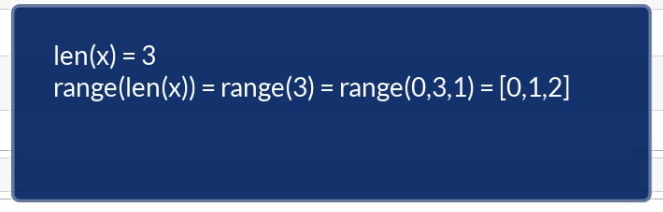### When to Use the Second Approach?

Honestly, both approaches can lead to the same outcome. Although the second one looks unnecessarily complicated, it might turn out to be a lot more useful In advanced coding. So, it is important to know both.

## Loops, Nested in Functions

We sometimes use loops in Python when we have to go through variables that are part of a list.

Now, let’s find out how to count the number of items whose value is less than 20 in a list.

First, we can define a function that takes numbers as an argument, where numbers will be a certain list variable. The trick is to create a variable that, so to speak, “departs” from 0. Let’s call it total.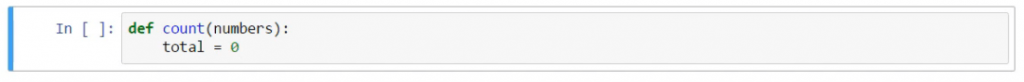The idea is that, when certain conditions are verified, total will change its value. This is why, in such a situation, it is appropriate to call this variable a rolling sum.

More technically, when we consider x in the numbers list, if it is smaller than 20, we will increment the total by 1 and finally return the total value. This means that:

1. if x < 20
• total will grow by 1
2. and if x >= 20
• total will not grow.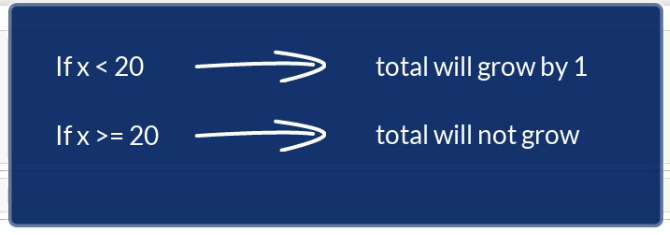So, for a given list, this count() function will return the amount of the numbers smaller than 20.

def count(numbers):
total = 0
for x in numbers:
if x<20:
total += 1
return total

Let’s confirm that this function works properly. We can create a list which has 4 numbers that are less than 20.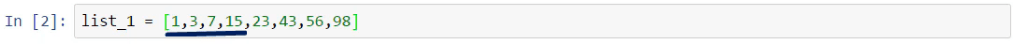As you can see in the picture below, it is working properly.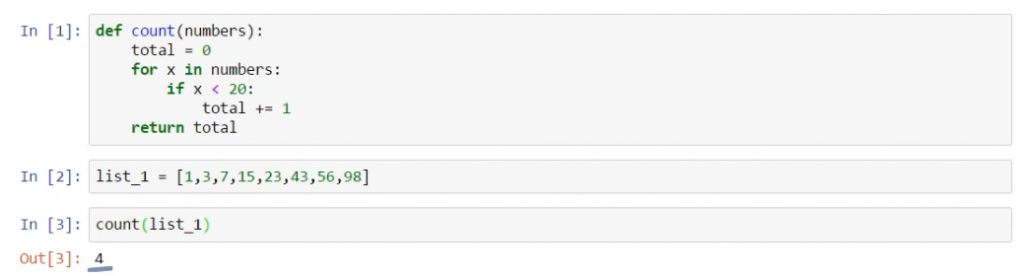Great! Now, if we add 17, for example, somewhere in the list, the outcome will adjust accordingly.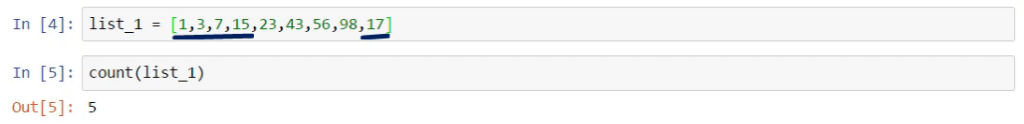Side note: Look how the whole if-statement is indented even more to the right. This allows us to separate it logically from the rest of the code in the cell that refers to this Python function.

## How to Iterate Through a Dictionary in Python

Because using loops in Python when working with dictionaries is not a piece of cake, let’s provide an example. We can create 2 dictionaries. The prices of a box of spaghetti, of a portion of lasagna, and of a hamburger are stored in a dictionary, called prices.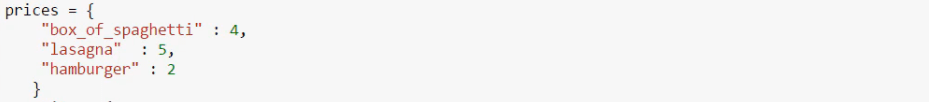Jan went to the supermarket and bought 6 boxes of spaghetti, 10 portions of lasagna, and no hamburgers. This data was stored in a dictionary, named quantity.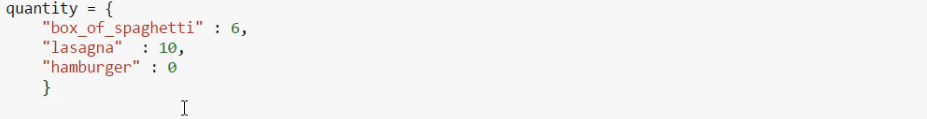Our problem is: how much did Jan spend in the supermarket? Well, it is obvious we’ll need to multiply the quantity of each food by its price.### Why Is It Easier to Use a Loop?

You must have noticed that our dictionaries have exactly the same keys. This is one of the strong points in our case and we should exploit this. The procedure:

1. Go to the box of spaghetti in the first dictionary
2. Take the value of 4
3. Get the value of 6 from the quantity dictionary
4. Multiply those two.

This operation must be repeated for each food product. This is exactly why it is easier to use a loop.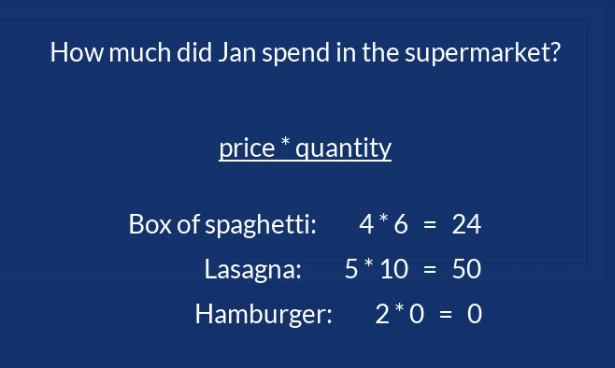Before anything else, some variable must account for the amount of money spent. Let’s implement a well-known trick. We can create a rolling sum, called money_spent, which will initially assume the value of 0.

So, we can start by iterating over each item in prices, i in prices for short.

money_spent = 0
for i in prices:

### The Body of the Loop

At every step of the loop, we would like the money_spent variable to grow by the product of the price and the quantity of a certain good i. We can achieve this by writing the following code:

for i in prices:
money_spent = money_spent + (prices[i] * quantity[i])

Let’s print the result to check whether we were working correctly.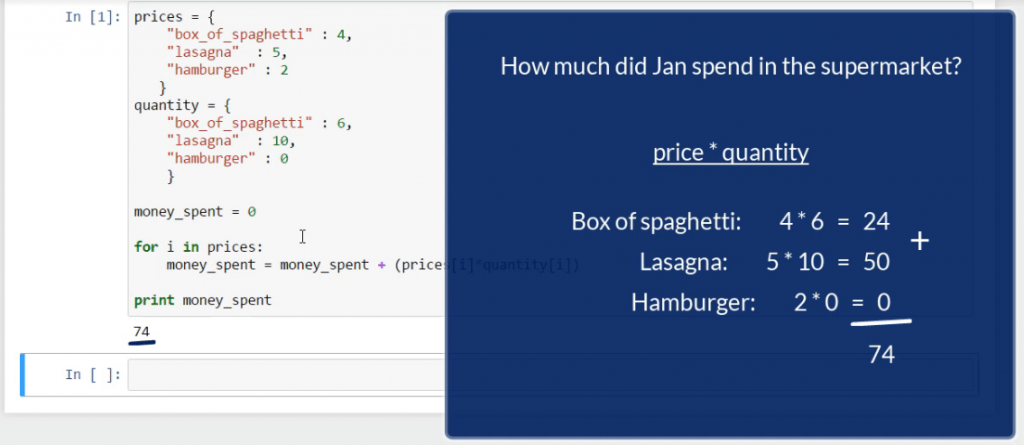Such a simple problem in terms of mathematics, required to connect knowledge on dictionaries, iteration, and creating a variable with an increment.

So, from a programmer’s perspective, the problem looks different. The good thing is that, in the end, this whole thing boiled down to a mere four lines of coding!

Side note: If we put quantity instead of prices at the beginning of the loop, the outcome will remain the same.

prices = {
“box_of_spaghetti” : 4,
“lasagna” : 5,
“hamburger” : 2
}

quantity = {
“box_of_spaghetti” : 6,
“lasagna” : 10,
“hamburger” : 0
}

money_spent = 0

for i in quantity:
money_spent = money_spent + (prices[i]*quantity[i])

print money_spent

It does not matter if you loop through prices or through quantity, because the two dictionaries contain the same keys. And this is the reason this loop works correctly, too.

## Why Do We Need Loops in Python?

To conclude, loops in Python will help you execute the same code until a given condition is not true anymore. Usually, both for loops and while loops are applicable to the same task – the for loop and the while loop. It mainly depends on your personal preferences which one to use. However, both of them have their advantages over the other in certain cases. In fact, now you know how to use both conditionals and functions alongside loops in Python.

If you are struggling over how you can convert real-life concepts into the world of programming, make sure you dive into the article about Object Oriented Programming. And, if you’re enthusiastic about boosting your Python knowledge, check out our super practical tutorials! Wondering which career paths in Python you can take? Find out in our Complete Python Programming Guide!